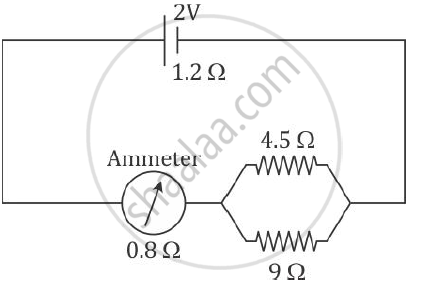Share

# A Cell of Emf 2 V and Internal Resistance 1.2 ω is Connected with an Ammeter of Resistance 0.8 Omega and Two Resistors of 4.5 Omega and 9 Omega as Shown in the Diagram Below: What Would Be the Reading on the Ammeter? - ICSE Class 10 - Physics

#### Question

A cell of Emf 2 V and internal resistance 1.2 Ω is connected with an ammeter of resistance 0.8 Ω and two resistors of 4.5 Ω and 9 Ω as shown in the diagram below:1) What would be the reading on the Ammeter?

2) What is the potential difference across the terminals of the cell?

#### Solution

Given that

ε = 2 V, r = 1.2 Ω, RA = 0.8 Ω, R1 = 4.5 Ω, R2 = 9 Ω

1) We know that for the circuit

ε = IRtotal

Now, the total resistance of the circuit is

R_"total" = r + R_A + R_p

1/R_p = 1/4.5 +   1/9 = 3/9

∴ Rp = 3 Ω

⇒ Rtotal = 1.2 + 0.8 + 3 = 6 Ω

Hence, the current through the ammeter is

I =  epsilon/R_"total" = 2/6 = 0.33 A

2) The potential difference across the terminals of the cell is V_"cell" = Ir = 0.33 xx 1.2 = 0.396 V

Is there an error in this question or solution?

#### APPEARS IN

Solution A Cell of Emf 2 V and Internal Resistance 1.2 ω is Connected with an Ammeter of Resistance 0.8 Omega and Two Resistors of 4.5 Omega and 9 Omega as Shown in the Diagram Below: What Would Be the Reading on the Ammeter? Concept: Internal Resistance.
S